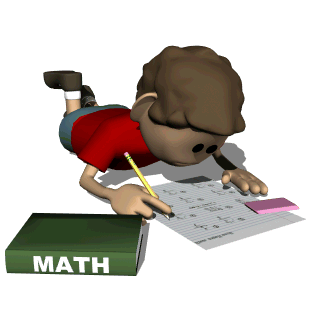• This is a list of Math Facts that your child is going to learn throughout the school year.
Practice makes perfect:)Doubles Facts
1+1=2
2+2=4
3+3=6
4+4=8
5+5=10
6+6=12
7+7=14
8+8=16
9+9=18
10+10=20
11+11=22
12+12=24

Almost Doubles
3+4=7
2+3=5
4+5=9
5+6=11
1+2=3
7+8=15
8+9=17
6+7=13
9+10=19

Ten Friends
3+7=10
4+6=10
1+9=10
5+5=10
6+4+10
7+3=10
9+1=10
8+2=10

Ten More
2+10=12
4+10=14
3+10=13
5+10=15
1+10=11
7+10=17
8+10=18
6+10=16
9+10=19
10+10=20

Nine More
2+9=11
4+9=13
3+912
5+9=14
1+9=10
7+9=16
8+9=17
6+9=15
9+9=18
10+9=19